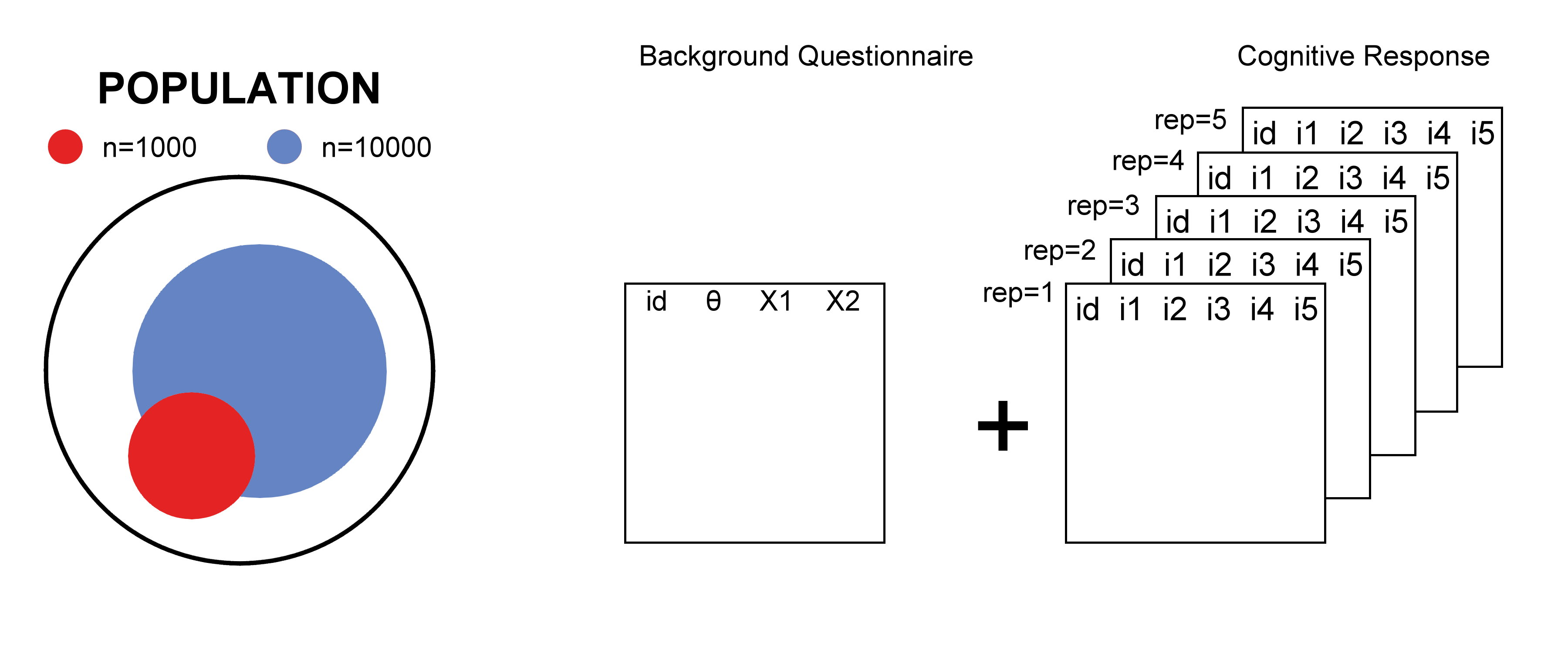Version 2.0.0 of the R package lsasim comes with new, interesting features for researchers working with background questionnaires. Of particular interest for this blog post is the ability to calculate the theoretical linear regression coefficients for the expectation of the latent trait $$\theta$$ on the answers to the $$Q$$ background questionnaire items. Mathematically, given the equation $E(\theta | Q) = \beta_0 + \sum_{i = 1}^q \beta_i Q_i,$ we’re interested in calculating $$\beta = \{\beta_1, \ldots, \beta_Q\}$$. This is done directly by the function beta_gen or, indirectly, by calling questionnaire_gen with the arguments theta = TRUE, full_output = TRUE, and family = "gaussian".

Since questionnaire_gen generates sample data from theoretical parameters such as the covariance matrix between $$\theta$$ and $$Q$$, estimates for $$\beta$$ can be calculated directly by applying linear regression—e.g. using the lm function available in base R—in the generated data. As a matter of fact, beta_gen uses this same covariance matrix to calculate the theoretical $$\beta$$. If cognitive responses are also available for those same students who wrote the background questionnaire, they can also be used by an IRT package such as TAM or mirt to estimate $$\beta$$.

An interesting exercise would be to check if those estimates for the regression coefficients match the parameters calculated by beta_gen. In order to reduce sample variability and get estimates which are as close as reasonably possible to the true values, one sample might not be enough, and a more complex simulation study must be setup. Then again, simply performing one experiment over and over is no guarantee that we will reach the expected results. In this post, we will show you how one apparently innocuous design decision can greatly affect the results.

# Simulation setup 1

Let a population of infinite size—in statistical terms—out of which an unbiased sample of students is drawn. In the figure below, the population is contained within the black circumference on the left, and two independent student samples are represented by the blue and red circles.The sampled students are then subjected to a background questionnaire and a cognitive questionnaire; their responses are graded and Item Response Theory—namely the TAM package—is used to estimate $$\theta$$ and $$\beta$$ from the cognitive responses.

Next, we administer another cognitive questionnaire to this same group of students. This seems to make sense at first, since the cognitive questionnaire is the only one being used by TAM to calculate the regression coefficients, and the student sample is an unbiased sample anyway. So a new cognitive questionnaire is administered, the answers are once again scored and $$\hat\beta$$ are once again estimated. This procedure of administering a new cognitive questionnaire is repeated a total of 1 000 times; those 1 000 estimates of $$\beta$$ are then compared with the true value. For starters, summary statistics for $$\hat\beta$$ in this setup are given in the tables below, which differ by the sample size (the table at the top results from a sample of 1 000 students; the one at the bottom results from a sample of 10 000).

N Variable Mean SD
1000 theta 0.0058 0.9827
1000 q1 -0.0132 1.0215
1000 q2 -0.0016 0.9858
<
N Variable Mean SD
10000 theta -0.0025 1.0005
10000 q1 0.0038 1.0115
10000 q2